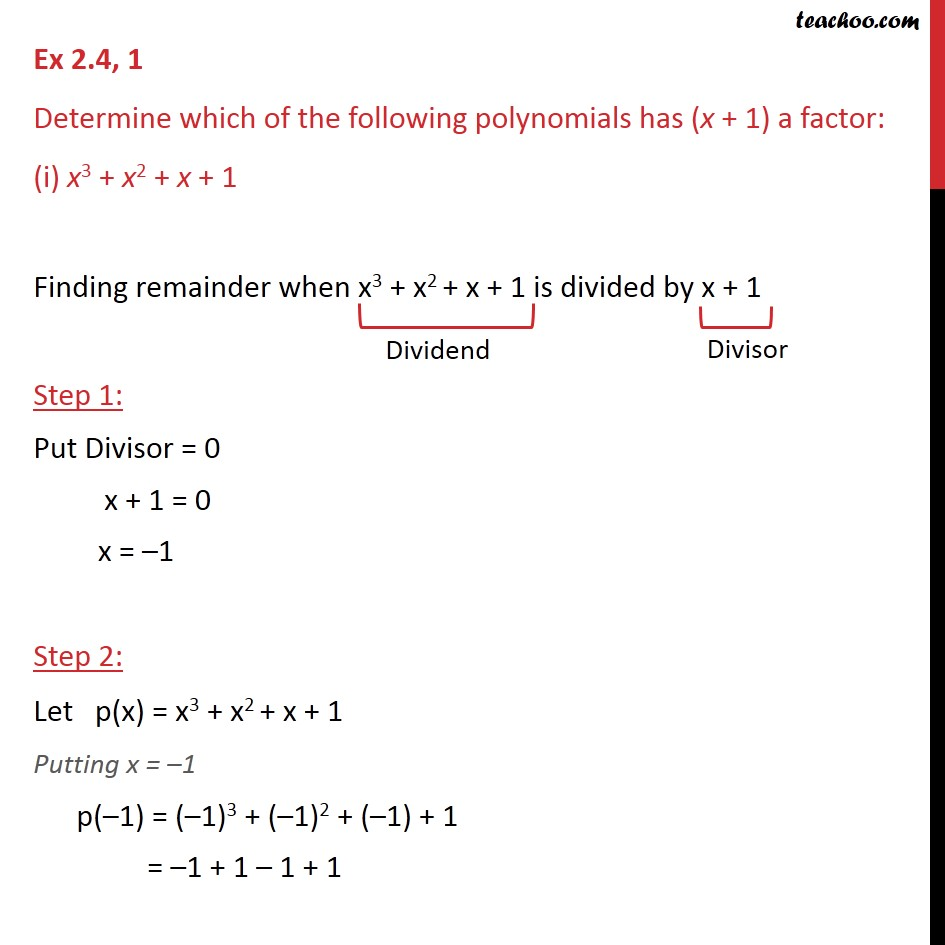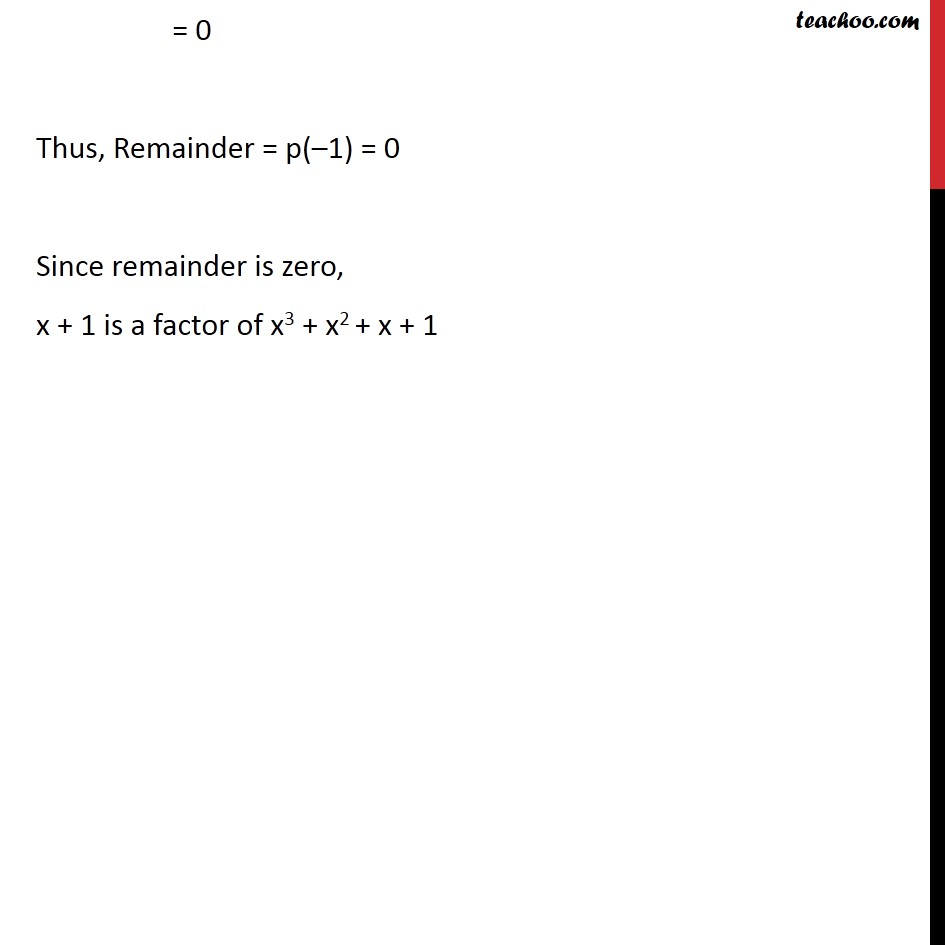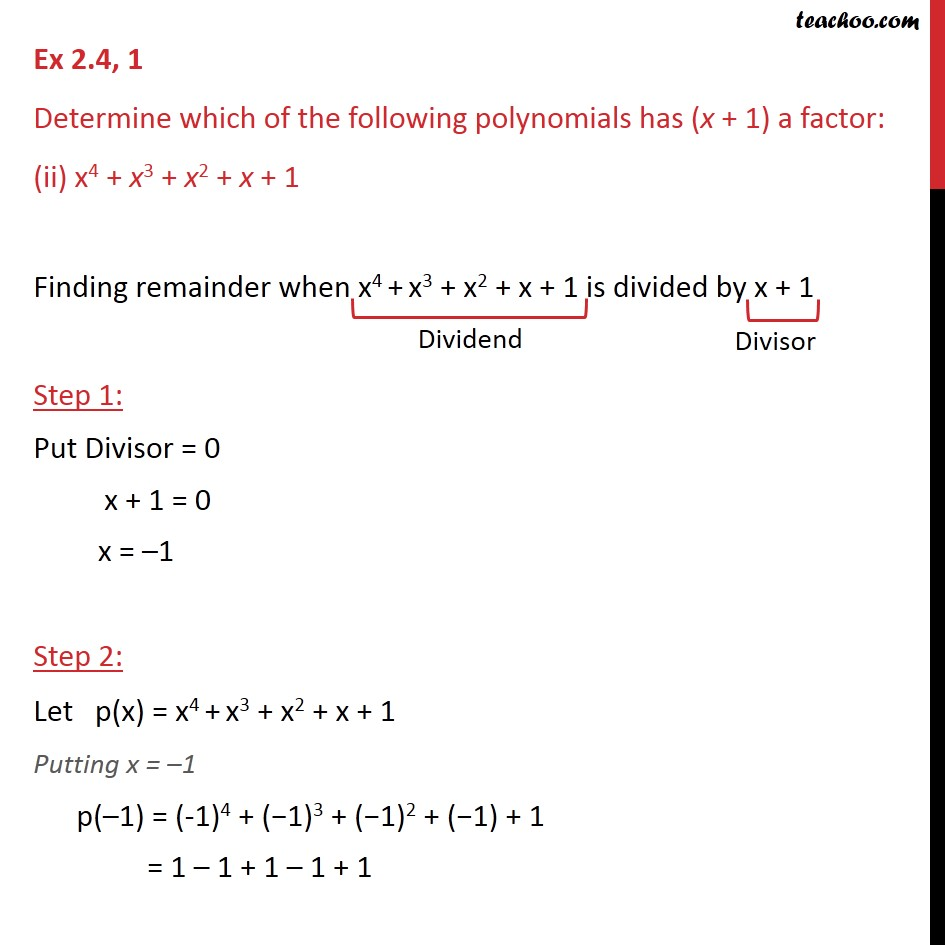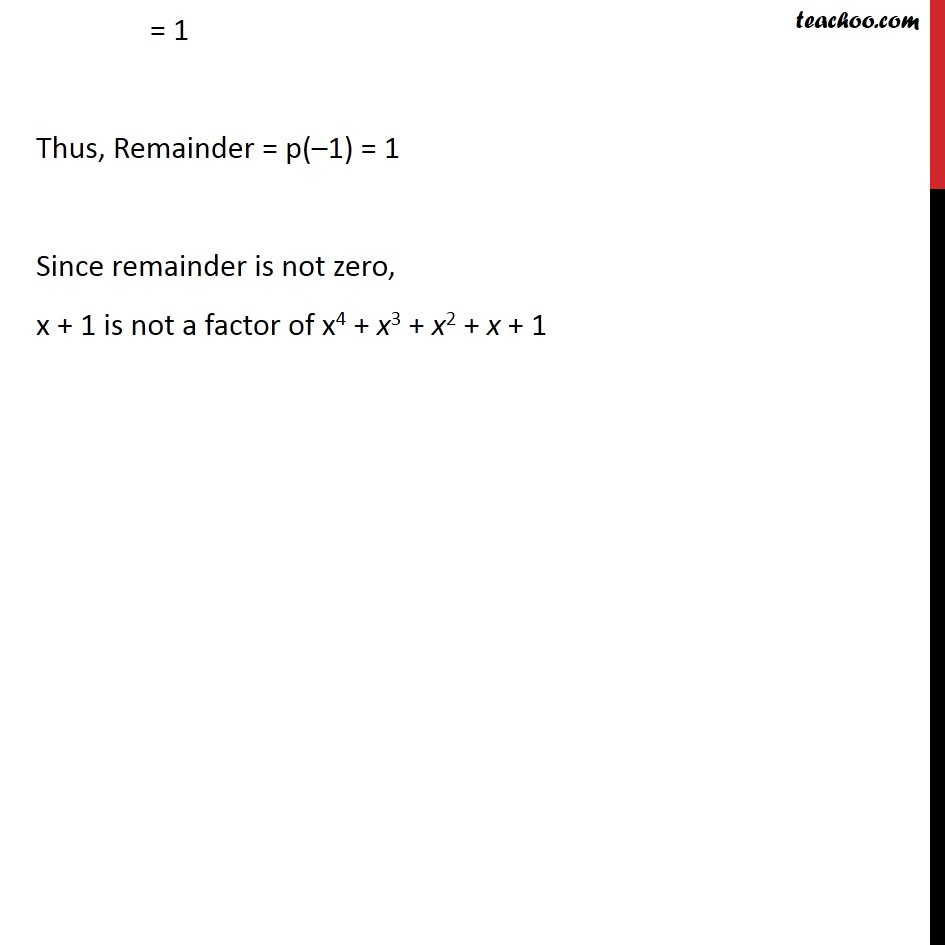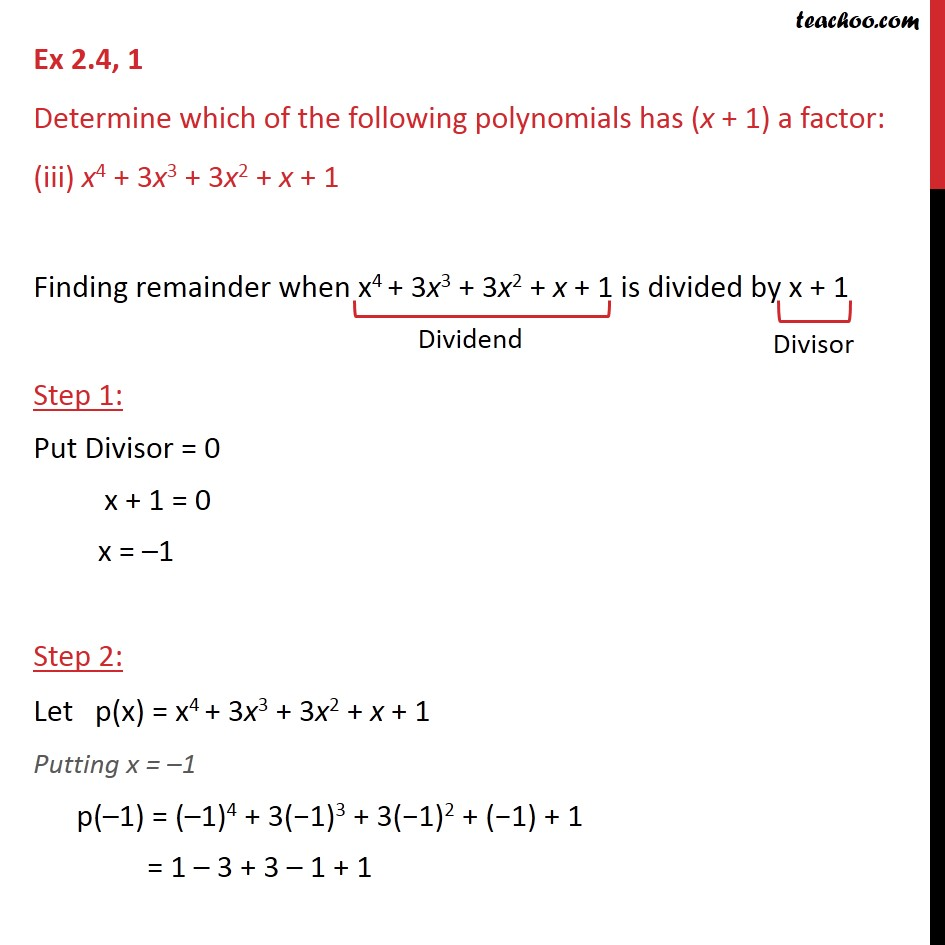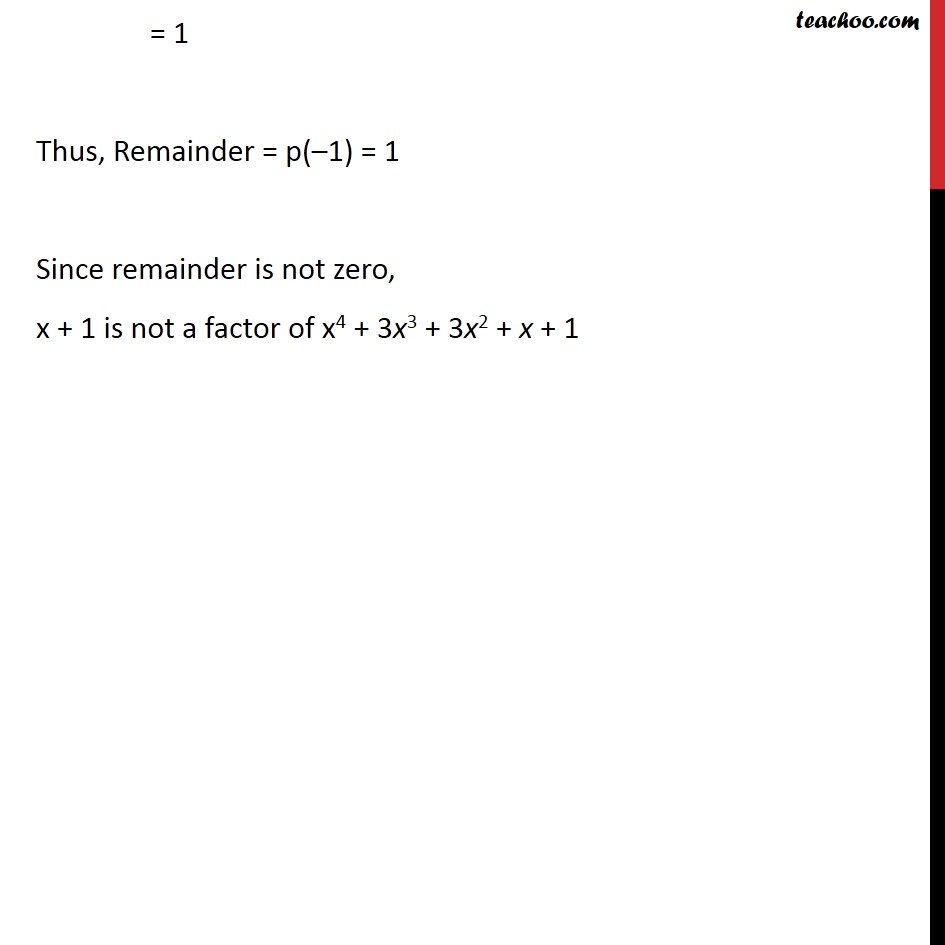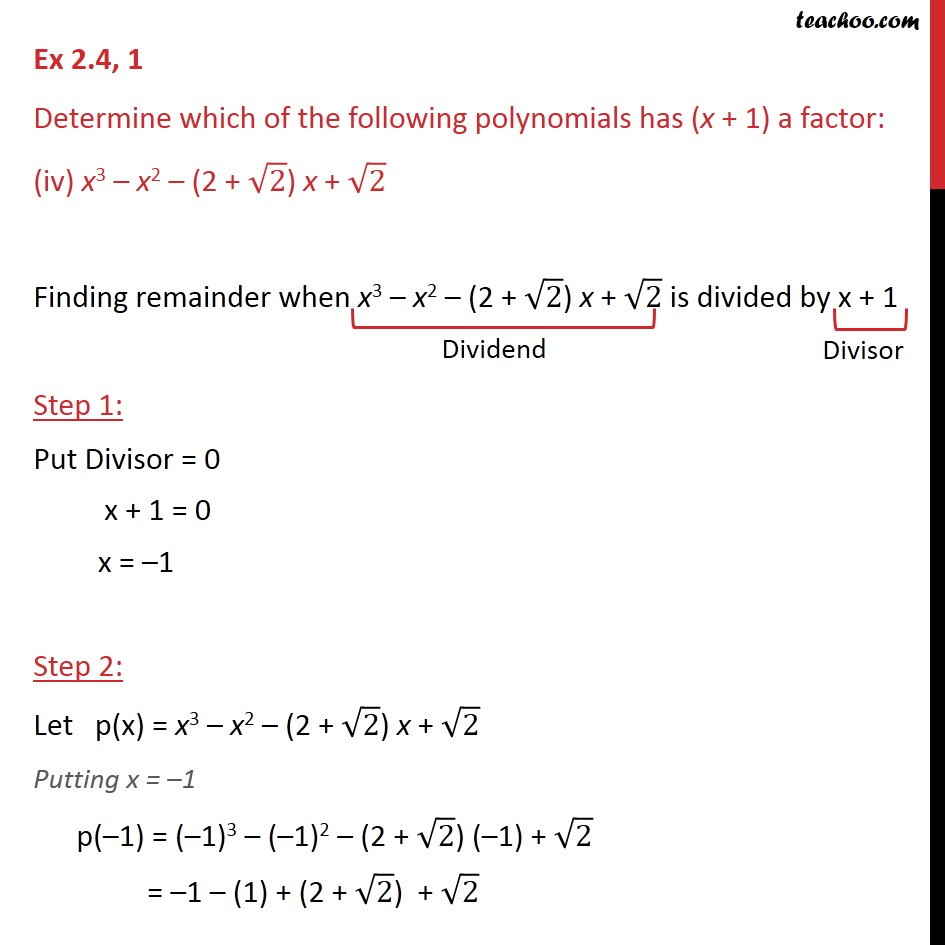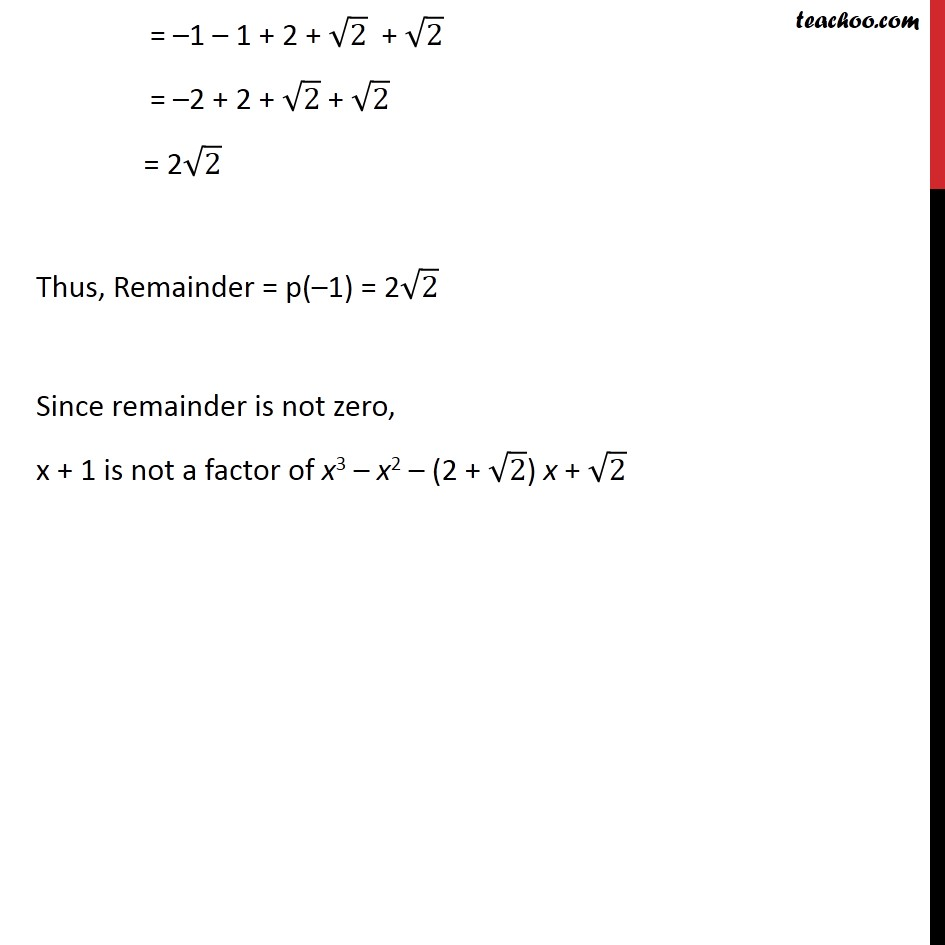1. Class 9
2. Important Questions for Exam - Class 9
3. Chapter 2 Class 9 Polynomials

Transcript

Ex 2.4, 1 Determine which of the following polynomials has (x + 1) a factor: (i) x3 + x2 + x + 1 Finding remainder when x3 + x2 + x + 1 is divided by x + 1 Step 1: Put Divisor = 0 x + 1 = 0 x = 1 Step 2: Let p(x) = x3 + x2 + x + 1 Putting x = 1 p( 1) = ( 1)3 + ( 1)2 + ( 1) + 1 = 1 + 1 1 + 1 = 0 Thus, Remainder = p( 1) = 0 Since remainder is zero, x + 1 is a factor of x3 + x2 + x + 1 Ex 2.4, 1 Determine which of the following polynomials has (x + 1) a factor: (ii) x4 + x3 + x2 + x + 1 Finding remainder when x4 + x3 + x2 + x + 1 is divided by x + 1 Step 1: Put Divisor = 0 x + 1 = 0 x = 1 Step 2: Let p(x) = x4 + x3 + x2 + x + 1 Putting x = 1 p( 1) = (-1)4 + ( 1)3 + ( 1)2 + ( 1) + 1 = 1 1 + 1 1 + 1 = 1 Thus, Remainder = p( 1) = 1 Since remainder is not zero, x + 1 is not a factor of x4 + x3 + x2 + x + 1 Ex 2.4, 1 Determine which of the following polynomials has (x + 1) a factor: (iii) x4 + 3x3 + 3x2 + x + 1 Finding remainder when x4 + 3x3 + 3x2 + x + 1 is divided by x + 1 Step 1: Put Divisor = 0 x + 1 = 0 x = 1 Step 2: Let p(x) = x4 + 3x3 + 3x2 + x + 1 Putting x = 1 p( 1) = ( 1)4 + 3( 1)3 + 3( 1)2 + ( 1) + 1 = 1 3 + 3 1 + 1 = 1 Thus, Remainder = p( 1) = 1 Since remainder is not zero, x + 1 is not a factor of x4 + 3x3 + 3x2 + x + 1 Ex 2.4, 1 Determine which of the following polynomials has (x + 1) a factor: (iv) x3 x2 (2 + 2) x + 2 Finding remainder when x3 x2 (2 + 2) x + 2 is divided by x + 1 Step 1: Put Divisor = 0 x + 1 = 0 x = 1 Step 2: Let p(x) = x3 x2 (2 + 2) x + 2 Putting x = 1 p( 1) = ( 1)3 ( 1)2 (2 + 2) ( 1) + 2 = 1 (1) + (2 + 2) + 2 = 1 1 + 2 + 2 + 2 = 2 + 2 + 2 + 2 = 2 2 Thus, Remainder = p( 1) = 2 2 Since remainder is not zero, x + 1 is not a factor of x3 x2 (2 + 2) x + 2

Chapter 2 Class 9 Polynomials

Class 9
Important Questions for Exam - Class 9